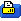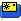Mar 01, 2012, 11:02 AM
Registered User
Discussion

# Thinking about induced drag, downwash

These questions were provoked as I was thinking about the long -running "shoved downward" thread.

Most of my reading in aerodynamics has had more to do with stability and control, I'm not sure I understand induced drag and downwash all that well.

As I understand it, induced drag is created by the difference between the relative wind (undisturbed flow) and the actual flow direction, as influenced by downwash. ( Like this http://en.wikipedia.org/wiki/Lift-in...f_induced_drag )

Consider this:

" Reducing induced drag

Theoretically a wing of infinite span and constant airfoil section would produce no induced drag. The characteristics of such a wing can be measured on a section of wing spanning the width of a wind tunnel, since the walls block spanwise flow and create what is effectively two-dimensional flow."

( http://en.wikipedia.org/wiki/Lift-in...g_induced_drag )

Is this really true?

Is such a test section, spanning the width of the tunnel, is there still a downwash behind the airfoil? Is the "effective relative airflow" still different than the free stream flow? Or not?

If yes, than how can there be no induced drag?

If no, then this test section is creating lift without turning air downwards?

Or maybe it's something like, in this ideal case with no tip vortices or infinite span, the downward-turning happens entirely behind the airfoil and doesn't affect the net flow around the airfoil itself?

In this diagram http://en.wikipedia.org/wiki/Lift-in...f_induced_drag , does the "effective relative airflow" represent a real flow direction that we could see with a tuft of yarn, or some sort of virtual flow representing the overall average effect of tip vortices etc-- ?

Now thinking about real-world winged flight out of the wind tunnel

If we put winglets on the wings to extract energy from the tip vortices, does this reduce the downward momentum of the downwash, as well as reducing the induced drag?

More generally-- so the airfoil is creating lift-- exerting a downward force on the air, as the air exerts an upward force on the airfoil-- is there a simple formula for the total amount of downward momentum imparted to the air?

Thinking about relationships between force, momentum, velocity, work, energy -- ?

In level flight, I suggest that it must be true that when the downwash eventually strikes the earth in whatever direct (ground effect) or non-direct form that takes, it imparts a downward force on the earth equal to the plane's weight, as argued in this thread "On the largest scale there usually is no downwash" https://www.rcgroups.com/forums/show....php?t=1602562.

Assuming for the moment that this is true, does knowing the magnitude of this downward force imparted by the downwash onto the earth (equal to the aircraft's weight) then give a simple way to calculate the downward momentum of the downwash? Or not?

Units of force -- Newtons
Units of momentum-- (mass times velocity) -- kg * m /s or N* s

When a plane flies in ground effect, so that induced drag is decreased, is the downward momentum of its downwash less than when the same plane flies out of ground effect? Yet the downward force ultimately imparted by the downwash to the ground is the same in both cases? Is this possible?

Consider a real airplane-- in ground effect, if you think it matters-- and then consider that we increase the aspect ratio more and more, tending toward infinite span. Does the momentum of the downwash tend toward zero? While the downward force imparted by the downwash on the earth stays constant? How can we describe in words what is going on here? Perhaps the downwash angle is getting shallower and shallower so that the downwash is spread out over a larger area? Or... ??

 Sign up now to remove ads between postsThread ToolsShow Printable VersionEmail this Page